# Physics - Electromagnetism - How to solve problems around CapacitorsSource

## Introduction

Hello it's a me again Drifter Programming!

Today we continue with Electromagnetism to get into how we solve problems that contain Capacitors and Capacitance.

All the mathematical equations will be drawn using quicklatex!

So, without further do, let's get straight into it!

## Capacitor combination

In my previous post I talked about capacitors and how we calculate the capacitance they have. The capacitance is connected with the physical properties of the capacitor and constant. This means that every time we want to use a capacitor for some application we search for one with the correct capacitance. But, most of the times one single capacitor is not enough and that's why we do combinations of capacitors.

Capacitors can be combinated with two main methods:

1. Series connection
2. Parallel connection

We of course can also have a mixed combination of those cases!

Let's get into each one and how we calculate the total and equivalent capacitance of the combination of those capacitors.

### Series connection

A series connection of capacitors looks like this: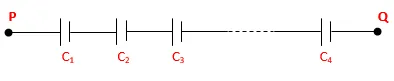Source

You can see that the capacitors are connected one after another and so in a serial way in between of two points P, Q that have a potential difference Vpq.

Supposing all the capacitors are at first uncharged and that Vpq is positive, the "upper" conductor plate of the Capacitor C1 will be charged with a positive charge Q. The electric field of the positive charge of this "upper" plate will attract the negative charges of the "other" plate of the same conductor and so this plate will be negatively charged. This of course means that this plate has a charge -Q. The negative charges of course come from the "upper" conductor of the next capacitor which is C2 and the "upper" plate of this capacitor will be positively charged with a charge +Q. By continuing with this procedure all the capacitors will have a positive charge +Q on top and -Q on the bottom conductive plate they build of.

Of course the bottom plate of a conductor Cn and the upper plate of the next capacitor let's say C(n+1) will always have a total charge zero!

We can clearly see that in serial connection the magnitude of charge of any capacitor is the same!

The potential difference of each capacitor is:
The total potential difference from the point P to Q is: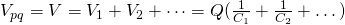That way we have:Last time we said that capacitance is given by: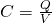By inverting this equation we of course get the previous result and so the total capacitance of capacitors connected with a serial connection is: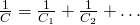The inverse of the equivalent capacitance of the serial combination of capacitors is equal to the sum of the inverse capacities of each capacitor.

### Parallel connection

A parallel connection looks like this:Source

Supposing all are uncharged and that the potential difference Vpq is again positive, of course all capacitors will have the same potential difference V = Vpq, cause they are connected parallely to the same endpoints P and Q. This means that we have a equipotential surface.

This time the charges are not equal and are given by;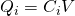The total charge is given by the sum:That way: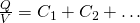Something which is equal to the capacitance of a capacitor and so the total capacitance of the parallel combination of capacitors is given by: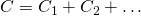The equivalent capacitance of the parallel combination of capacitors is equal to the sum of the capacities of each capacitor.

Note that:

• In parallel connection the total-equivalent capacity is always larger than any individual capacity.
• In serial connection the total-equivalent capacity is always smaller than any individual capacity.

## How to solve problems around Capacitors

To solve problems that contain Capacitors you should always remember the following:

• When a capacitor has charge Q we mean that the positive conductor it's build of has charge +Q (>0) and that the negative conductor has -Q.
• In a serial connection the capacitors have the same charge Q when starting of uncharged. The potential differences are unequal (Vi) and the total potential difference is equal to the sum each individual potential difference (V = V1 + V2 + ...).
• In a parallel connection the capacitors have the same potential difference V. The charges (Qi) of each capacitor are unequal if the capacities (Ci) are unequal. The total charge Q is equal to the sum of each individual charge ( Q = Q1 + Q2 + ...).
• In mixed combinations we define sections that are simple serial or parallel combinations and replace them step by step with the equivalent capacities. That way we simplify the problem of calculating the total equivalent capacity of a mixed combination. When having to calculate the potential difference or charge of some specific capacitor then you might have to do this reductive approach in the opposite direction!

## Simple example

Let's lastly also get into a simple example of a mixed combination.

Suppose we have the following mixed combination: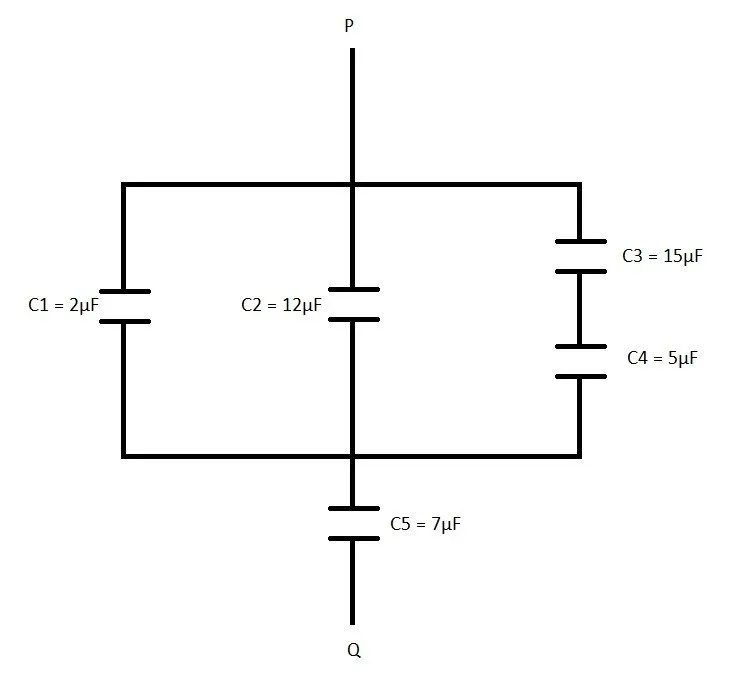Calculate the total equivalent capacitance!

We can see that C3 and C4 that are serially connencted.

The total capacitance C3, 4 of them is:
That way we now have the following diagram:
Now C1, C2 and the equivalent C3,4 capacity are in a parallel connection.

This means that the equivalent of them is equal to the sum of each individual capacity and so: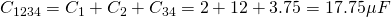That way we now get the following diagram: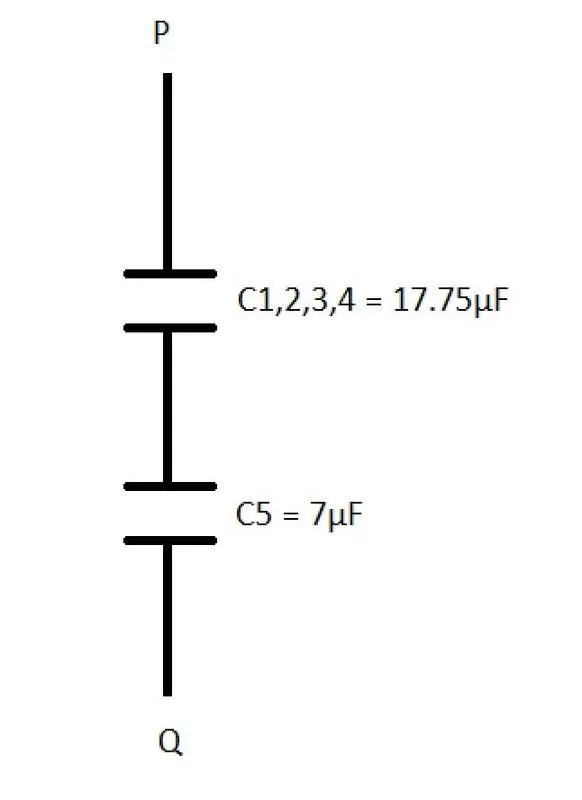Here the equivalent C1234 and C5 are now in serial connection which means that the final equivalent capacity is calculated using:So, the final diagram is:
Note that all the diagrams where made by myself :)

Intro

Physics Introduction -> what is physics?, Models, Measuring

Vector Math and Operations -> Vector mathematics and operations (actually mathematical analysis, but I don't got into that before-hand :P)

Classical Mechanics

Velocity and acceleration in a rectlinear motion -> velocity, accelaration and averages of those

Rectlinear motion with constant accelaration and free falling -> const accelaration motion and free fall

Rectlinear motion with variable acceleration and velocity relativity -> integrations to calculate pos and velocity, relative velocity

Rectlinear motion exercises -> examples and tasks in rectlinear motion

Position, velocity and acceleration vectors in a plane motion -> position, velocity and accelaration in plane motion

Projectile motion as a plane motion -> missile/bullet motion as a plane motion

Smooth Circular motion -> smooth circular motion theory

Plane motion exercises -> examples and tasks in plane motions

Force and Newton's first law -> force, 1st law

Mass and Newton's second law -> mass, 2nd law

Newton's 3rd law and mass vs weight -> mass vs weight, 3rd law, friction

Applying Newton's Laws -> free-body diagram, point equilibrium and 2nd law applications

Contact forces and friction -> contact force, friction

Dynamics of Circular motion -> circular motion dynamics, applications

Object equilibrium and 2nd law application examples -> examples of object equilibrium and 2nd law applications

Contact force and friction examples -> exercises in force and friction

Circular dynamic and vertical circle motion examples -> exercises in circular dynamics

Electromagnetism

Getting into Electromagnetism -> electromagnetim, electric charge, conductors, insulators, quantization

Coulomb's law with examples -> Coulomb's law, superposition principle, Coulomb constant, how to solve problems, examples

Electric fields and field lines -> Electric fields, Solving problems around Electric fields and field lines

Electric dipoles -> Electric dipole, torque, potential and field

Electric charge and field Exercises -> examples in electric charges and fields

Electric flux and Gauss's law -> Electric flux, Gauss's law

Applications of Gauss's law (part 1) -> applying Gauss's law, Gauss applications

Applications of Gauss's law (part 2) -> more Gauss applications

Electric flux exercises -> examples in electric flux and Gauss's law

Electric potential energy -> explanation of work-energy, electric potential energy

Calculating electric potentials -> more stuff about potential energy, potential, calculating potentials

Millikan's Oil Drop Experiment -> Millikan's experiment, electronvolt

Cathode ray tubes explained using electric potential -> cathode ray tube explanation

Electric potential exercises (part 1) -> applications of potential

Electric potential exercises (part 2) -> applications of potential gradient, advanced examples

Capacitors (Condensers) and Capacitance -> Capacitors, capacitance, calculating capacitance

And this is actually it for today!

Next time we will talk about Electric field energy!

Bye!

H2
H3
H4
3 columns
2 columns
1 column